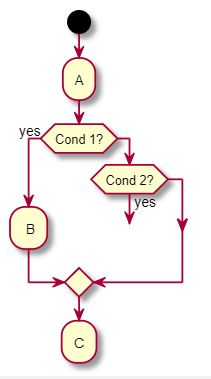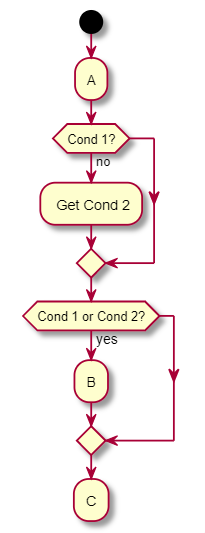# How to join two if paths in Activity Diagram Beta Syntax

How can I create this minimal example diagram with the new beta syntax for activity diagrams. I could not figure out on how to join the two conditional outputs (C1 yes, C2 yes) into one node (B):

@startuml

(*) --> "A"

if "Cond 1?" then

--> [yes] B

else

if "Cond 2?" then

--> [yes] B

else

--> [no] C

endif

endif

--> C

@enduml

answered Dec 12, 2019 by (12,020 points)

Hello,

What you try to di is a "goto". The beta syntax is really near code. I answered to a similar question in https://forum.plantuml.net/10278/interlacing-conditions

commented Dec 13, 2019 by (120 points)

```@startuml
start
:A;
if (Cond 1?) then (yes)
label labB
: B;
else
if (Cond 2?) then (yes)
goto labB
endif
endif
:C;
@enduml```

it renders like this. Am I using it wrong or is this a bug in the beta.commented Dec 13, 2019 by (120 points)

I thought about it again. If I would have to program it would probably rather this below which can be used as alternative yet make the diagram more complex. However I would be interested in the status of to 'label', 'goto' syntax.

```@startuml
start
:A;
if (Cond 1?) then (no)
: Get Cond 2;
endif
if (Cond 1 or Cond 2?) then (yes)
:B;
endif
:C;
@enduml```